I'm still learning. (Michelangelo, age 87)

Email: mnaim [at] mit [dot] edu

I am a currently an ICoN Postdoctoral Fellow at MIT working with Guangyu Robert Yang using AI to model the brain. I have a strong background in physics and math, and a profound passion for AI, especially in the areas of language and memory.

Previously, I finished my Ph.D. at the Weizmann Institute of Science under supervision of Misha Tsodyks. During my Ph.D. I spent some time at Institute for Advanced Studies and at Kavli Institute for Theoretical Physics.

Before that, I obtained my Bachelor's and Master's degrees in Physics at La Sapienza under supervision of Giorgio Parisi and Alessandro Treves (SISSA).

I am deeply committed to AI safety and its responsible advancement. I am particularly interested on the study of mechanistic interpretability and inner alignment in neural networks. I aim to make AI more understandable, believing that this will help us learn and grow faster.

This website doesn't describe my current research, but it's related to things I thought about and would like to share.

# Nature project: Clementines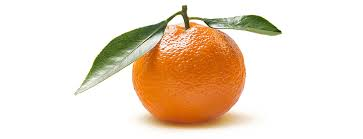In Italy, during Christmas time, we play a game called tombola (similar to the game of bingo). The player purchases cards based on a fixed cost decided at the start of the game. Each card contains 15 random numbers (between 1 and 90). Whenever the dealer draws a number that is present on one or more of their cards, the player "covers" the corresponding number. Usually numbers are covered with beans, chickpeas, lentils or other material available after Christmas dinners such as clementine skin. The ultimate goal of the game is to make "tombola", that is, to be the first player to cover all the numbers on one of their cards.

In 2014, while playing tombola, I asked myself my first research question: "How many segments are in a clementine?". Since that day, with the help of other people, I started collecting data for fun. In 2017, I had an excel sheet with 10898 different samples.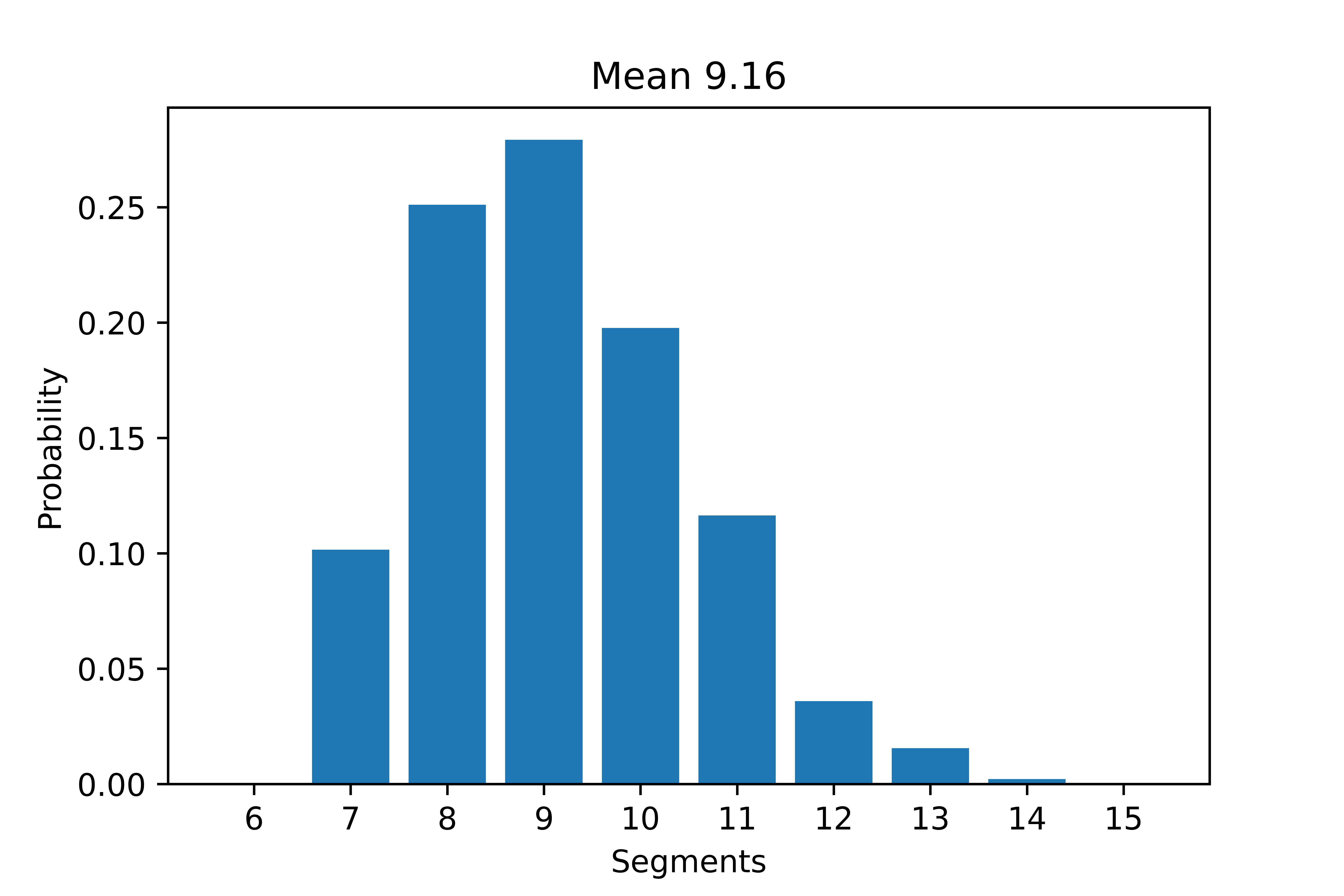# Math conjecture: High-water marks

While working on my Ph.D., I encountered an interesting problem related to the high-water marks. Given a sequence of values $${a_k}_{k=1}^n$$, the high-water marks are the values at which the running maximum increases. For example, given a sequence $$[3,5,7,8,8,5,7,9,2,5]$$ with running maxima $$[3,5,7,8,8,8,9,9,9]$$, the high-water marks are $$[3,5,7,8,9]$$. For every sequence $$a_k$$ there is a number of high-water marks $${N}_{a_k}$$.

If now we consider a set $$\sigma$$ of all permutations of $$n$$ numbers $$(1, \dots, n)$$ what is the analytical expression for the distribution of the number of high-water marks $$N_{a\in \sigma}$$? For example, if $$n=3$$, the distribution vector is $$(\frac{2}{3!},\frac{3}{3!},\frac{1}{3!})$$.

To solve this problem, consider the cycle representation of a permutation (including the trivial one-element cycles). We have a bijection of the symmetric group with itself that maps between numbers of cycles and numbers of high-water marks. Thus the distribution of the number of high-water marks is the distribution of the number of cycles. This is given by the unsigned Stirling numbers of the first kind, which has a distribution $$P_{s} (k;n) = \frac{|s(n;k)|}{n!} \, ,$$ where $$\begin{cases} s(n;1) = (n-1)! \\ s(n;n-1) = \frac{n(n-1)}{2} \\ s(n;n) = 1 \end{cases}$$

For the full proof (special thanks to Jeroen Zuiddam) you can click here.

# Math riddle

Imagine you have a bucket of water containing two distinct types of bacteria: A and B. You start with one bacterium of each type, A and B. Every time you introduce a particle of food into the bucket, one of the bacteria consumes it. The bacterium that eats the food reproduces, adding one more bacterium of its type to the population. If you were to drop 100 particles of food into the bucket, can you predict the distribution of the A-type bacteria?

Example: Suppose the bucket contains 3 A-type bacteria and 2 B-type bacteria. If you drop a food particle and an A-type bacterium consumes it, the population changes to 4 A-type bacteria and 2 B-type bacteria. On the other hand, if a B-type bacterium eats the food, the population becomes 3 A-type and 3 B-type bacteria.

The simulation above is a hint and represents the outcome after 9 drops of food. It runs 1,000 times to generate a histogram that showcases the probability distribution of the number of A-type bacteria.

# Mice maze game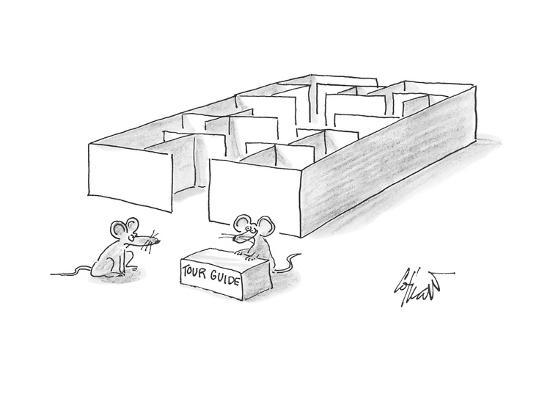Mazes have been a staple of animal psychology for well over 100 years. Rosenberg et al. (2021) presented a new approach to the study of learning and decision-making in mice. They give the animal access to a complex labyrinth and leave it undisturbed for a night while monitoring its movements. The result is a rich data set that reveals new aspects of learning and the structure of exploratory behavior.

Inspired by this paper, I decided to build a simple game (using GameMaker Studio2) that allows you to experience the same labyrinth as the mice did.

Instructions: The goal of the game is to recover a treasure inside a maze and return to the starting room. The faster you go back, the higher the score. Use the arrow keys to move the character.

# AI: some exercises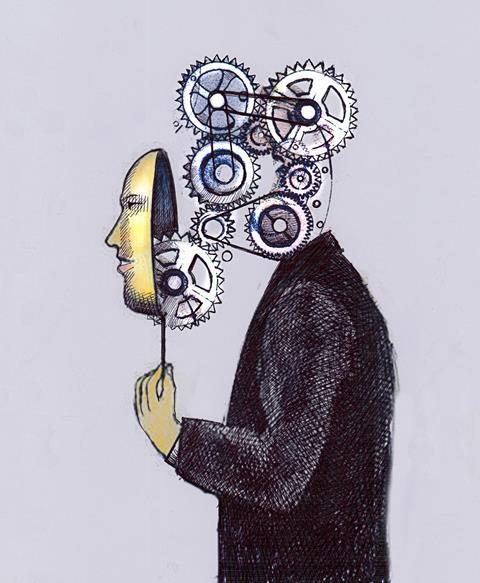Do you know how to code and you are passionate about AI? Did you try to play with it but lost motivation because "too hard"? Would like to better understand what exactly it is about and be able to apply it in your own research? Great, this post is for you!!

Here is a small set of exercises that will walk you through some of the basics of AI. These exercises will help you better familiarize with PyTorch, fully connected networks (FC), convolutional neural networks (CNN), graph neural networks (GNN), attention mechanism and reinforcement learning (RL).

# AI project: 2048

2048 is a game played on a plain 4x4 grid, with numbered tiles that slide when a player moves them using the four arrow keys. Every turn, a new tile randomly appears in an empty spot on the board with a value of either 2 or 4. Tiles slide as far as possible in the chosen direction until they are stopped by either another tile or the edge of the grid. If two tiles of the same number collide while moving, they will merge into a tile with the total value of the two tiles that collided. The resulting tile cannot merge with another tile again in the same move. The game is won when a tile with a value of 2048 appears on the board. Players can continue beyond that to reach higher scores. When the player has no legal moves (there are no empty spaces and no adjacent tiles with the same value), the game ends.

The AI algorithm is an evolutionary algorithm. In 2048 you can get pretty far by just pressing random keys. Therefore, building an AI is much harder, since the difference between random movements and intelligence movements is very small. With the help of an algorithm, the AI will construct future game states and pick the one that has a higher score. The score is computed taking into account not only the highest number, but also adjacent blocks of the same number and having the highest number on the corner.

Now look at the AI as it learns :)

# AI project: Chess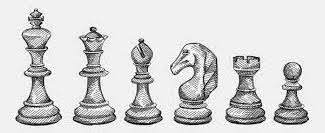AI has influenced the way in which chess games are played at the top level. In order to build a simple AI that can play chess we are going to use an algorithm called minimax. In this algorithm there are two players, player A that tries to reach the highest value possible (max), while player B is trying to reach the lowest value possible (min).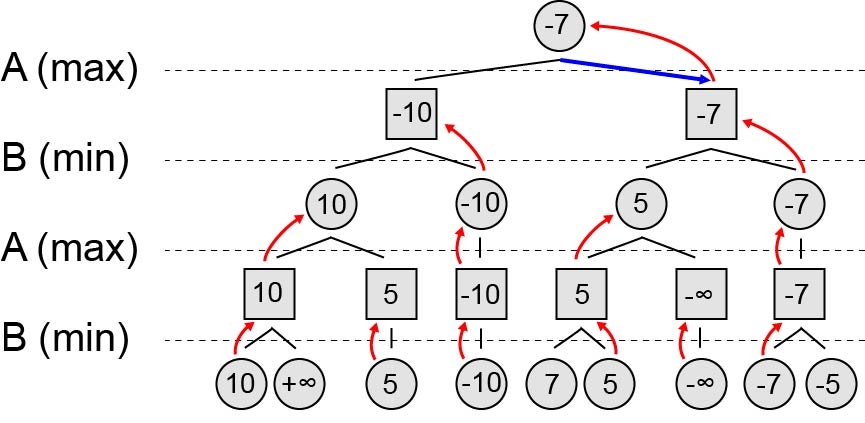Let's consider a tree (figure above) where players alternate in making moves. Assuming that player B is perfectly logical and places the best move possible, then which move should you make first as player A? The trick is to work backward. If player B was at each intersection on the bottom layer, which direction would they choose? For the bottom left intersection they would choose 10 as it is the lower of the two. That means the A player should consider the value of that intersection to be 10. We do this for all the bottom intersections, then we look one layer up and think if player A was at each of these intersections which action would they take. We repeat this procedure until finally we will be left with two choices: either go left (-10) or right (-7). Therefore, to maximize their score, A should pick right!

Chess is just a big game of minimax. We can create a score giving value to all the pieces (Pawn = 1, Knight = 3, Bishop = 3, Rook = 5, Queen = 9) and then adding all the white pieces and subtracting all the black pieces. From white prespective, you are trying to reach a high schore, while black wants to reach a low score. The AI needs to generate all the boards that are possible to reach from our current one within a certain number of moves, then calculate the score and find the best possible move it can take.

# AI project: Minesweeper

Minesweeper is a game where mines are scattered throughout a board, which is divided into cells. Cells have three states: unopened, opened and flagged. An unopened cell is blank and clickable, while an opened cell is exposed. Flagged cells are those marked by the player to indicate a potential mine location. A player selects a cell to open it. If a player opens a mined cell, the game ends. Otherwise, the opened cell displays either a number, indicating the number of mines diagonally and / or adjacent to it, or a blank tile, and all adjacent non-mined cells will automatically be opened.

The problem with minesweeper is that at "hard" is a game of luck. Even the best player can get stuck with a 50% of loosing. This AI is not a neural network, rather some coding. First few moves are random, then it uses some logic:

1. If a cell has the same amount of hidden squares around it as unflagged bombs remaining around it, then all the hidden cells are bombs.
2. If a cell has the same amount of flags around it as its number, then all the hidden cells are not bombs.
3. Using probability the AI is able to select cells that have a lower chance of being bombs. Let's deifine $$N_i$$ as the number of bombs arrangements in which a bomb is placed on a cell $$i$$, and $$T$$ as the total number of possible bomb arrangements in the remaining cells. Now we have that the probability of cell $$i$$ to be a bomb is given by $$P_i = \frac{N_i}{T} \, .$$

Now look at the AI as it plays. If the AI wins the number of bombs increases by 1 (with a limit of 25 bombs).

# Fantasy Shares

Since Italy did not qualify for the World Cup 2022, I organized a simple game in order to follow the tournament having fun throughout all the 64 games.

The idea is pretty simple: each player has some credits that are used to buy "shares" of the different teams (1 share = 1 credit), without knowing which shares the other players own. Every time a team wins a match, it gets a certain number of points. These points are divided among the team shareholders, according to the number of shares they own.# Poetry

Sometimes, I love to read and write poetry. Here are a few I would like to share:

1. I always want eyes (in hebrew) - December 2019

2. You, Israel (in hebrew) - May 2020

3. Estate (in italian) / Summer (in english) - July 2022

4. Albero (in italian) - May 2023

# Beliefs

I believe in the axioms by Federico Ardila:

• Axiom 1. Mathematical talent is distributed equally among different groups, irrespective of geographic, demographic, and economic boundaries.

• Axiom 2. Everyone can have joyful, meaningful, and empowering mathematical experiences.

• Axiom 3. Mathematics is a powerful, malleable tool that can be shaped and used differently by various communities to serve their needs.

• Axiom 4. Every student deserves to be treated with dignity and respect.